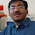Source: Quantnet Interview Questions

Problem:
If a car passes at the crosswalk on average every 10 seconds and you need 20 seconds to pass the road, how long does it take you on average to cross the road?

Note that since the problem is not very well specified, make reasonable assumptions to solve it. It would be fun if before solving mathematically, you try and guess a time estimate. :-)

Update (3rd January 2011):
Solution posted by Santosh Ananthakrishnan (EE Final Year DD Student IIT Bombay) and Animesh Saxena (Manager at Karvy Private Wealth) in comments!

1.154.650769 ?? I can't say its unreasonable.

Assumed exponential distribution for inter arrival times. Couldn't use any intuition.

2.If we assume that arrivals are Poisson, then I think we get a nice recursive formulation -

Suppose the first arrival occurs at t, then the expected waiting time T can be written as:
1. If t > 20s, it is just 20s.
2. If t < 20s, it is t + T, because the process is Poisson.

But we know the distribution of t -
P(no arrivals in t) = e^(-lambda*t) where lambda is the parameter of the arrival process, here 1/10.

So P(some arrivals before t)= 1-e^(-lambda*t)

and the distribution for an arrival at t is lambda*e^(-lambda*t)

Putting all that together,

T= 20*(prob of no arrivals in 20s)
+ (average t+T over 0-20s)

= 20*e^(-1/10*20) + integral(t = 0 to 20)(1/10 * e^(-t/10) * (t + T) dt)

One part of the integral works out to (1 - e^(-2))T, so the RHS becomes e^(-2)T, and on the LHS, we're left with

20*e^(-2) + integral(t = 0 to 20)(1/10 * e^(-t/10) * t dt ).

That integral evaluates to
t*e^(-t/10) + 10*e^(-t/10) from 20 to 0, which is

10 - 20*e^(-2) - 10*e^(-2).

So the RHS is
= 10 - 10*e^(-2)

The LHS was e^(-2)*T,

so finally,
T = 10*(e^2) - 10
= 63.89 seconds, a bit more than 1 minute.

3.10*(e^2-1). I checked the quantnet solution and realized I made a series mistake.
Anyways I did it conditioning on the number of cars you would encounter before crossing.

= Sum{ (20+nT)*(cdf(20))^n(1-cdf(20))}

where T is the expected time for a car to arrive conditioned on time<20 seconds.

T evaluates to 10*(e^2-3)/(e^2-1) and the series sums to 10*(e^2-1).

4.This one seems similar to birds on wire problem. Assuming arrival of cars as Poisson distribution, we can calculate the exp value...so lambda is 0.1 or 1/10...integrating using intervals 0 to 20 and 20 to infinity...the answer comes to approx 64

5.Yes, the answer is approximately 64. (As pointed out by Santosh and Animesh). Thanks